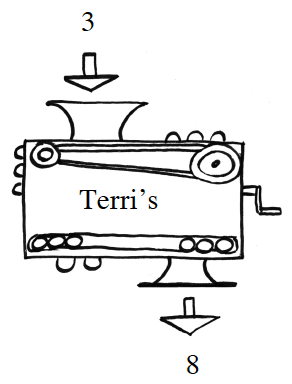### Home > CCA2 > Chapter Ch1 > Lesson 1.1.2 > Problem1-14

1-14.

Terri’s project for the Math Fair was a magnificent black box that she called a function machine. If you put $3$ into her machine, the output would be $8$. If you put in $10$, the output would be $29$; and if you put in $20$, it would be $59$.

1. What would her machine do to the input $5$? What about $−1$? What about $x$? Making an input → output table may help.

Look for a pattern.

Try making a table and graphing the points.

If the input is $x$, what is the output?

$\left. \begin{array} { | c | c | c | c | c | c | } \hline x & { 3 } & { 10 } & { 20 } & { 5 } & { - 1 } \\ \hline 3 x - 1 & { 8 } & { 29 } & { 59 } & { 14 } & { - 4 } \\ \hline \end{array} \right.$

2. Write an equation for Terri’s machine.

$f(x)=3x−1$